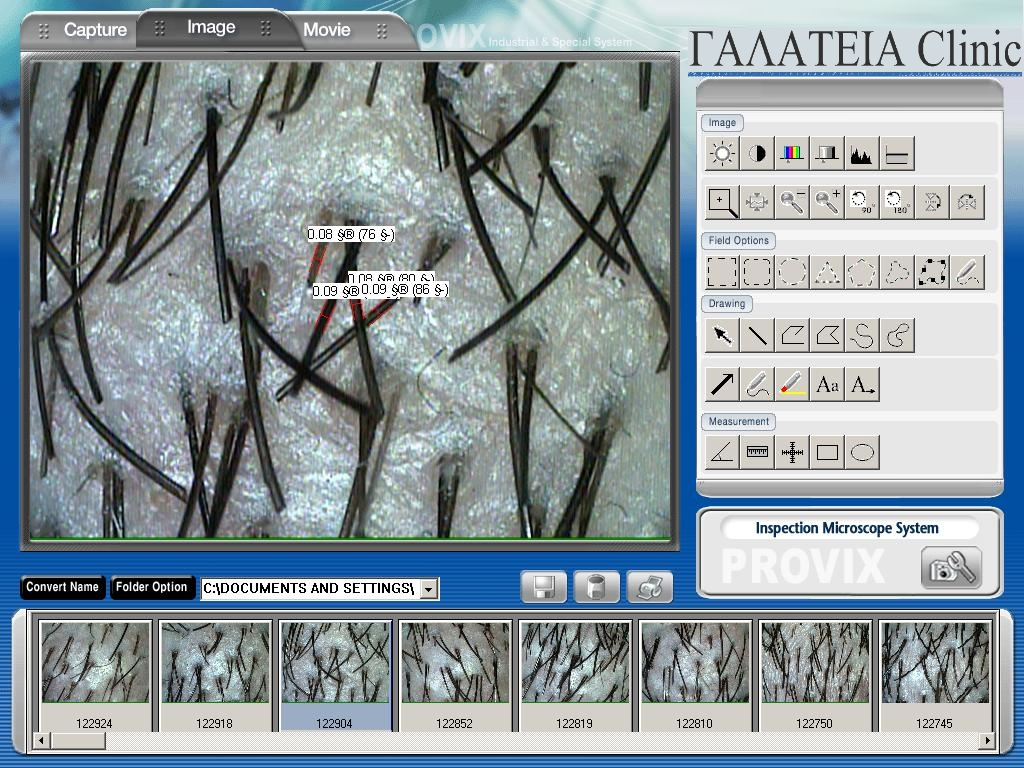Calculating the necessary amount of grafts

Calculating the necessary amount of micrografts is a very important part of planning the hair transplantation procedure. Achieving the desired result depends on this. The calculation is based on a mathematical principle that comprises the following parametres:

• The number of follicular units per square centimetre in the zones that are not affected by hair loss
• The number of hairs per square centimetre in the zones not affected by hair loss
• The diametre of the hairs in the zones not affected by hair loss
• The number of follicular units per square centimetre in the zones affected by hair loss
• The number of hairs per square centimetre in the zones affected by hair loss
• The diametre of the hairs in the zones affected by hair loss
• The surface area of the zones

Measuring the above-listed parametres is done by studying the scalp with a microcamera that provides precise information: